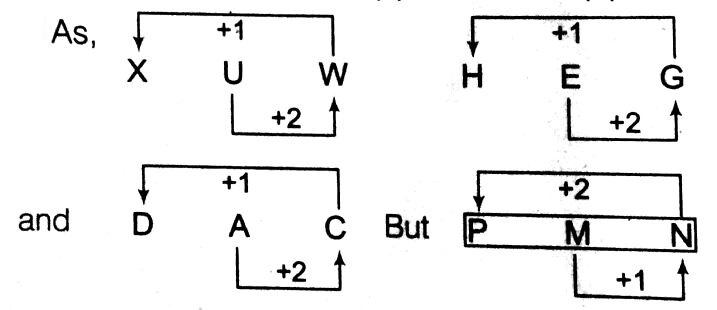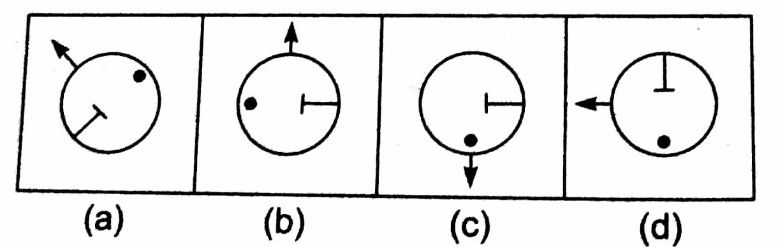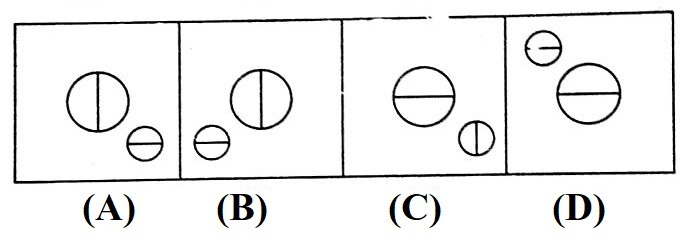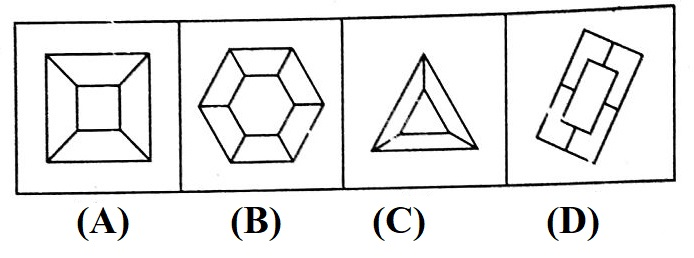# Practice Classification Questions and Answers

## Classification

Classification is an important part of the Reasoning syllabus of various competitive exams like- SSC CGL, SSC CHSL and many others. It is a very scoring topic as it does not involve any calculations. Let us know in detail what exactly can you expect from the topic of ‘Classification’.

### What is Classification?

• In simple language, to ‘classify’ means to assign some peculiar characteristic to various members/part of a group and identify them on the basis of those peculiar characteristics only.
• Questions on Classification are meant to test candidates' skill in determining the common characteristics among the given set of words/images/numbers.
• So, the candidates will be required to find out the word/image/number which is least like the word/image/number given in the question.

The most important thing to note while solving questions on Classification is that the peculiar feature must be unique and must not be present in the odd option. Moreover, candidates must also have a basic awareness of general knowledge including Science, Geography, Mathematics, etc.

### Finding the Odd-Word

In these types of questions, four meaningful words will be given. Out of these four words, three words will have similar features and based on that the candidates are required to find out the odd-word.

For example-

Q1) FiInd the odd word.

• Cholera
• Tetanus
• Smallpox
• Typhoid

Explanation:

All except ‘smallpox’ are diseases caused by bacteria, while ‘small pox’ is caused by viruses.

### Finding the Odd-Pair of Words

Here, four options will be given. Each option has a pair of words and out of these four  pairs, three pairs will bear a common relationship. The candidates will be required to find out the odd-pair which does not share that common relationship.

For instance-

Q1) Find the odd pair.

• Lion: Roar
• Snake: Hiss
• Frog: Bleat
• Bees: Hum

Explanation:

In the pair of Frog: Bleat, bleat is the sound made by sheep and not frogs. Hence, option (c) is the correct answer.

### Finding the Odd Letters/Numbers

Here, four options will be given containing a random group of letters/numbers. Out of these four, three of these random groups of letters/numbers will have a common pattern and the candidates are required to figure out the option which does not share the common pattern.

For example-

Q1) Find the odd letters.

• XUW
• PMN
• HEG
• DAC

Explanation:Hence, the term ‘PMN’ is different from the rest.

Q2) Find the odd number.

• 356
• 639
• 527
• 246

Explanation:

Except ‘356,’ in all the other numbers the sum of the first two digits is equal to the third digit of the number.

For solving questions on finding the odd numbers, you must keep a following things in mind-

• The basic mathematical operations i.e., addition, subtraction, multiplication and division.
• The family of numbers i.e., even, odd, prime and rational etc.
• Patterns and divisibility test.
• Square and cube of numbers.
• Square root and cube root of numbers.
• Place values of alphabetical letters.

### Finding the Odd Figure

In such types of questions, four figures will be given in the options. Among them, three will belong to a common group and one will not belong to that group. The candidates are required to find the odd figure from the given options.

For example-

Q1) FInd the odd figure.Explanation:

In figure, (c) the dot point ‘●’ is to the left of ‘T’, while in other dot point ‘●’ appears to be the opposite of ‘T’.

While solving questions on finding the odd figures, you must keep a following things in mind-

• The structure of figures and elements
• The rotation of elements.
• The distribution of elements.
• The position and placing of elements.
• Increasing or decreasing level of elements.

### Sample Questions on Classification

Q1) Find the odd letter.

• Treachery
• Fraud
• Deceit
• Morbid

Explanation:

In the above question, all the words except Morbid are synonymous to each other as they all mean ‘falsehood’. However, the word ‘morbid’ means ‘diseased’

Q2) In the following question, select the one which is different from the other three responses.

• Shimla
• Darjeeling
• Ooty
• Agra

Explanation:

Except ‘Agra’, all others are mountain places.

Q3) Find the odd Number/letters from the given alternative.

• BE
• GJ
• NP
• QR

Explanation:

G(7) + J(10) → 17 (odd)

N(14) + P(16) → 30(even)

Q(17) + R(18) → 35(odd)

Hence, Option (c) is an odd option.

Direction: In each of the following questions, out of four, three are similar in a certain manner, one figure is different from the other three. Choose a figure which is different from others.

Q4)Explanation:

Except figure (d) all others are divided in four parts, but figure (d) is divided in eight parts.

Q5)Explanation:

Except figure (d) the middle design of other figures are the same and bottom figure is the water image of the top figure.

Q6) Find the odd pair.

• Needle – Prick
• Gun – Fire
• Auger – Bore
• Chisel – Carve